# Invertible sheaf

(diff) ← Older revision | Latest revision (diff) | Newer revision → (diff)

A locally free sheaf of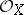-modules of rank 1 on a ringed space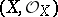. An equivalent definition is: A sheaf of-modules that is locally isomorphic to the sheaf. The invertible sheaves on, considered up to isomorphism, form an Abelian group with respect to the operation of tensor multiplication over. This group is called the Picard group of the space, and is denoted by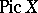. The inverse of a sheaf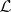in this group is the sheafdual to. In the case whenis a scheme (in particular, an algebraic variety) or an analytic space, a sheaf of-modules is invertible if and only if it is isomorphic to the sheaf of regular (respectively, analytic) sections of some algebraic (respectively, analytic) line bundle over.

Invertible sheaves on schemes are closely connected with divisors (cf. Divisor). With each Cartier divisoronis associated an invertible sheaf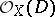, thus defining an injective homomorphism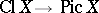, whereis the group of classes of Cartier divisors on. For integral schemesthis homomorphism is an isomorphism.

On a projective schemeSerre's twisted invertible sheafcan be defined. In fact, if an imbedding of the schemein a projective space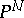is given, thencorresponds to the class of a hyperplane section. In particular, ifis a projective space over a field, then the sheaf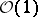is the direct image of the sheaf of linear functions onunder the natural mapping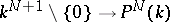. The system of homogeneous coordinatesin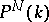can be identified with a basis for the space of sections.

An invertible sheaf on a schemeis related to rational mappings ofinto projective spaces. Letbe an invertible sheaf on a scheme and let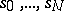be sections ofthe values of which at any pointgenerate the stalk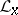over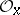. Then there exists a unique morphism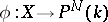such that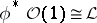and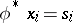, where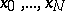are homogeneous coordinates in. An invertible sheaf onis called very ample if there exists an imbeddingsuch that. An invertible sheafonis called ample if there exists a positive integerfor which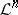is very ample. On a Noetherian schemeoveran invertible sheafis ample if and only if for each coherent sheaf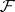onthere exists an integersuch that the sheaf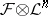is generated by its global sections for.

Ifis an ample invertible sheaf oncorresponding to a divisor, thenis called an ample divisor. A Cartier divisoron a schemethat is proper and smooth over an algebraically closed fieldis ample if and only if for each closed integral subscheme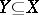the intersection index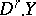is positive, where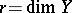(cf. Intersection index (in algebraic geometry)). For other criteria of ampleness see . There is also a generalization of the concept of an ample divisor on subvarieties of large codimension .

The concepts of very ample and ample invertible sheaves can be carried over to the case of analytic spaces (for criteria for ampleness in this situation see Positive vector bundle).

How to Cite This Entry:
Invertible sheaf. Encyclopedia of Mathematics. URL: http://encyclopediaofmath.org/index.php?title=Invertible_sheaf&oldid=12066
This article was adapted from an original article by V.A. Iskovskikh (originator), which appeared in Encyclopedia of Mathematics - ISBN 1402006098. See original article